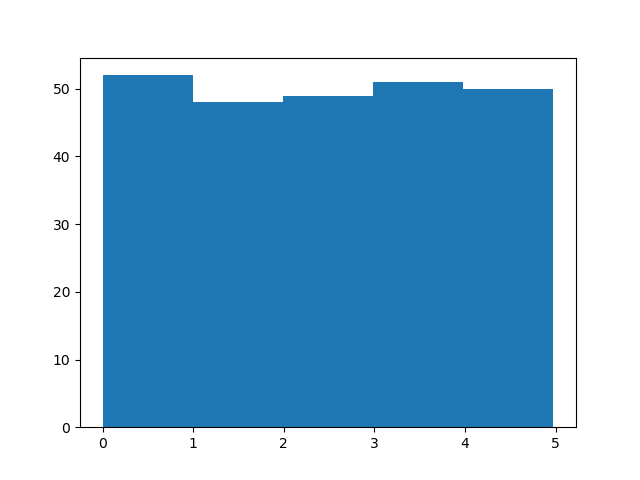# 机器学习 - 数据分布

## 数据分布（Data Distribution）

### 实例

```import numpy

x = numpy.random.uniform(0.0, 5.0, 250)

print(x)
```

## 直方图

### 实例

```import numpy
import matplotlib.pyplot as plt

x = numpy.random.uniform(0.0, 5.0, 250)

plt.hist(x, 5)
plt.show()
```

### 结果：### 直方图解释

```52 values are between 0 and 1
48 values are between 1 and 2
49 values are between 2 and 3
51 values are between 3 and 4
50 values are between 4 and 5
```

## 大数据分布

### 实例

```import numpy
import matplotlib.pyplot as plt

x = numpy.random.uniform(0.0, 5.0, 100000)

plt.hist(x, 100)
plt.show()
```